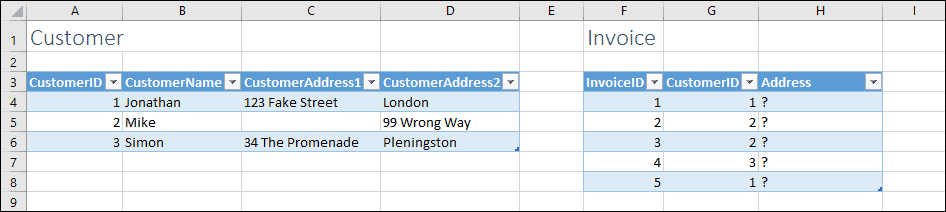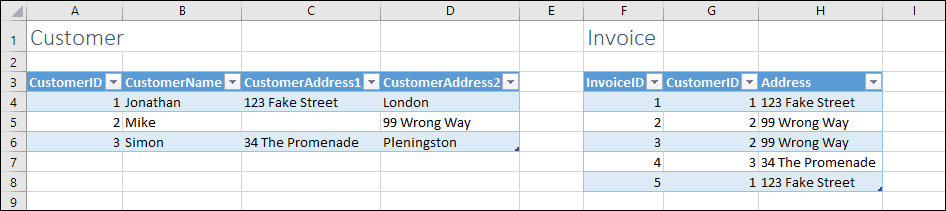# Using VLOOKUP, if Column 1 is blank, get value from Column 2

Here’s a mockup example that I created:You want to use VLOOKUP to extract the first line of the address into column H, but you want your formula to return the address from column D if the value in column C is blank.

## Solving the problem with the IF function

The IF function allows you to solve this problem, though IF functions can be difficult to understand.

The IF and VLOOKUP functions are both fully explained in our Expert Skills Books and E-books.

The formula to solve this problem is:

=IF(VLOOKUP([@CustomerID],Customer,3)=””,VLOOKUP([@CustomerID],Customer,4),VLOOKUP([@CustomerID],Customer,3))

This formula works as follows:

IF the value in the 3rd column of the Customer table is blank, return the value in the 4th column of the Customer table, otherwise return the 3rd column of the Customer table.

## Sample Workbook

You can download a copy of the sample workbook to see the formulas in action.Scroll to Top